# 使用 Azure 机器学习对模型进行超参数优化

1. 定义参数搜索空间
2. 指定要优化的主要指标
3. 为低性能运行指定提前终止策略
4. 创建和分配资源
5. 使用所定义的配置启动试验
6. 将训练运行可视化
7. 为模型选择最佳配置

## 什么是超参数优化？

“超参数优化”（也称为“hyperparameter optimization”）是找到用于获得最佳性能的超参数配置的过程。 通常，该过程在计算方面成本高昂，并且是手动的。

Azure 机器学习使你能够自动执行超参数优化，并且并行运行试验以有效地优化超参数。

## 定义搜索空间

### 离散超参数

• 一个或多个逗号分隔值
• `range` 对象
• 任意 `list` 对象
``````    {
"batch_size": choice(16, 32, 64, 128)
"number_of_hidden_layers": choice(range(1,5))
}
``````

• `quniform(low, high, q)` - 返回类似于 round(uniform(low, high) / q) * q 的值
• `qloguniform(low, high, q)` - 返回类似于 round(exp(uniform(low, high)) / q) * q 的值
• `qnormal(mu, sigma, q)` - 返回类似于 round(normal(mu, sigma) / q) * q 的值
• `qlognormal(mu, sigma, q)` - 返回类似于 round(exp(normal(mu, sigma)) / q) * q 的值

### 连续超参数

• `uniform(low, high)` - 返回高低之间的均匀分布值
• `loguniform(low, high)` - 返回根据 exp(uniform(low, high)) 绘制的值，使返回值的对数均匀分布
• `normal(mu, sigma)` - 返回正态分布的实际值，包括平均值 μ 和标准方差 σ
• `lognormal(mu, sigma)` - 返回根据 exp(normal(mu, sigma)) 绘制的值，使返回值的对数呈正态分布

``````    {
"learning_rate": normal(10, 3),
"keep_probability": uniform(0.05, 0.1)
}
``````

### 超参数空间采样

• 随机采样
• 网格采样
• 贝叶斯采样

#### 随机采样

``````from azureml.train.hyperdrive import RandomParameterSampling
from azureml.train.hyperdrive import normal, uniform, choice
param_sampling = RandomParameterSampling( {
"learning_rate": normal(10, 3),
"keep_probability": uniform(0.05, 0.1),
"batch_size": choice(16, 32, 64, 128)
}
)
``````

#### 网格采样

``````from azureml.train.hyperdrive import GridParameterSampling
from azureml.train.hyperdrive import choice
param_sampling = GridParameterSampling( {
"num_hidden_layers": choice(1, 2, 3),
"batch_size": choice(16, 32)
}
)
``````

#### 贝叶斯采样

Bayesian 采样仅支持搜索空间中的 `choice``uniform``quniform` 分布。

``````from azureml.train.hyperdrive import BayesianParameterSampling
from azureml.train.hyperdrive import uniform, choice
param_sampling = BayesianParameterSampling( {
"learning_rate": uniform(0.05, 0.1),
"batch_size": choice(16, 32, 64, 128)
}
)
``````

## 指定主要指标

• `primary_metric_name`：主要指标的名称需要与训练脚本记录的指标的名称完全匹配。
• `primary_metric_goal`：可以是 `PrimaryMetricGoal.MAXIMIZE``PrimaryMetricGoal.MINIMIZE`，确定在评估运行时是要将主要指标最大化还是最小化。
``````primary_metric_name="accuracy",
primary_metric_goal=PrimaryMetricGoal.MAXIMIZE
``````

### 记录用于超参数优化的指标

``````from azureml.core.run import Run
run_logger = Run.get_context()
run_logger.log("accuracy", float(val_accuracy))
``````

## 指定提前终止策略

• `evaluation_interval`：应用策略的频率。 每次训练脚本都会将主要指标计数记录为一个间隔。 如果 `evaluation_interval` 为 1，则训练脚本每次报告主要指标时，都会应用策略。 如果 `evaluation_interval` 为 2，则会每隔一次应用该策略。 如果未指定，则默认将 `evaluation_interval` 设置为 1。
• `delay_evaluation`：将第一个策略评估延迟指定的间隔数。 这是一个可选参数，可让所有配置运行最小间隔数，避免训练运行过早终止。 如果已指定，则每隔大于或等于 delay_evaluation 的 evaluation_interval 倍数应用策略。

Azure 机器学习支持以下提前终止策略：

### 老虎机策略

• `slack_factor``slack_amount`：在最佳性能训练运行方面允许的松驰。 `slack_factor` 以比率的形式指定允许的松驰。 `slack_amount` 以绝对数量（而不是比率）的形式指定允许的松驰。

例如，假设以间隔 10 应用老虎机策略。 另外，假设性能最佳的运行以间隔 10 报告了主要指标 0.8，目标是最大化主要指标。 如果策略指定的 `slack_factor` 为 0.2，则间隔为 10 时其最佳指标小于 0.66 (0.8/(1+`slack_factor`)) 的任何训练运行都会被终止。

• `evaluation_interval`：（可选）应用策略的频率

• `delay_evaluation`：（可选）将第一个策略评估延迟指定的间隔数

``````from azureml.train.hyperdrive import BanditPolicy
early_termination_policy = BanditPolicy(slack_factor = 0.1, evaluation_interval=1, delay_evaluation=5)
``````

### 中间值停止策略

• `evaluation_interval`：应用策略的频率（可选参数）。
• `delay_evaluation`：将第一个策略评估延迟指定的间隔数（可选参数）。
``````from azureml.train.hyperdrive import MedianStoppingPolicy
early_termination_policy = MedianStoppingPolicy(evaluation_interval=1, delay_evaluation=5)
``````

### 截断选择策略

• `truncation_percentage`：要在每个评估间隔终止的性能最低的运行百分比。 一个介于 1 到 99 之间的整数值。
• `evaluation_interval`：（可选）应用策略的频率
• `delay_evaluation`：（可选）将第一个策略评估延迟指定的间隔数
``````from azureml.train.hyperdrive import TruncationSelectionPolicy
early_termination_policy = TruncationSelectionPolicy(evaluation_interval=1, truncation_percentage=20, delay_evaluation=5)
``````

### 无终止策略（默认设置）

``````policy=None
``````

### 选择提前终止策略

• 对于既可以节省成本又不会终止有前景的作业的保守策略，请考虑使用 `evaluation_interval` 为 1 且 `delay_evaluation` 为 5 的中间值停止策略。 这属于保守的设置，可以提供大约 25%-35% 的节省，且不会造成主要指标损失（基于我们的评估数据）。
• 如果想节省更多成本，则可以使用老虎机策略或截断选择策略，因为前者的可用可宽延时间更短，后者的截断百分比更大。

## 创建和分配资源

• `max_total_runs`：最大训练运行数。 必须是介于 1 到 1000 之间的整数。
• `max_duration_minutes`：（可选）超参数优化试验的最长持续时间（分钟）。 此持续时间之后的运行会被取消。

• `max_concurrent_runs`：（可选）可以并发运行的最大运行数。 如果未指定此项，所有运行都将并行启动。 如果指定了此项，则必须是 1 和 100 之间的整数。

``````max_total_runs=20,
max_concurrent_runs=4
``````

## 配置超参数优化试验

• 所定义的超参数搜索空间
• 你的提前终止策略
• 主要指标
• 资源分配设置
• ScriptRunConfig `script_run_config`

ScriptRunConfig 是将通过采样的超参数运行的训练脚本。 它定义每个作业的资源（单节点或多节点）和要使用的计算目标。

`script_run_config` 中使用的计算目标必须具有足够的资源来满足你的并发级别。 有关 ScriptRunConfig 的详细信息，请参阅配置训练运行

``````from azureml.train.hyperdrive import HyperDriveConfig
from azureml.train.hyperdrive import RandomParameterSampling, BanditPolicy, uniform, PrimaryMetricGoal

param_sampling = RandomParameterSampling( {
'learning_rate': uniform(0.0005, 0.005),
'momentum': uniform(0.9, 0.99)
}
)

early_termination_policy = BanditPolicy(slack_factor=0.15, evaluation_interval=1, delay_evaluation=10)

hd_config = HyperDriveConfig(run_config=script_run_config,
hyperparameter_sampling=param_sampling,
policy=early_termination_policy,
primary_metric_name="accuracy",
primary_metric_goal=PrimaryMetricGoal.MAXIMIZE,
max_total_runs=100,
max_concurrent_runs=4)
``````

`HyperDriveConfig` 设置传递给 `ScriptRunConfig script_run_config` 的参数。 `script_run_config` 反过来将参数传递给训练脚本。 以上代码片段取自示例笔记本使用 PyTorch 进行训练、超参数优化和部署。 在此示例中，将优化 `learning_rate``momentum` 参数。 运行的提前停止将由 `BanditPolicy` 决定，它将停止一个主要指标落在 `slack_factor` 之外的运行（请参阅 BanditPolicy 类引用）。

``````# from pytorch_train.py
def main():
print("Torch version:", torch.__version__)

# get command-line arguments
parser = argparse.ArgumentParser()
help='number of epochs to train')
default=0.001, help='learning rate')
args = parser.parse_args()

print("data directory is: " + data_dir)
model = fine_tune_model(args.num_epochs, data_dir,
args.learning_rate, args.momentum)
os.makedirs(args.output_dir, exist_ok=True)
torch.save(model, os.path.join(args.output_dir, 'model.pt'))
``````

## 提交超参数优化试验

``````from azureml.core.experiment import Experiment
experiment = Experiment(workspace, experiment_name)
hyperdrive_run = experiment.submit(hd_config)
``````

## 热启动超参数优化（可选）

• 贝叶斯采样：来自先前运行的试用将用作先验知识来选取新样本，并改善主要指标。
• 随机采样网格采样：提前终止使用从以前的运行获得的知识来确定性能不佳的运行。

``````from azureml.train.hyperdrive import HyperDriveRun

warmstart_parent_1 = HyperDriveRun(experiment, "warmstart_parent_run_ID_1")
warmstart_parent_2 = HyperDriveRun(experiment, "warmstart_parent_run_ID_2")
warmstart_parents_to_resume_from = [warmstart_parent_1, warmstart_parent_2]
``````

``````from azureml.core.run import Run

resume_child_run_1 = Run(experiment, "resume_child_run_ID_1")
resume_child_run_2 = Run(experiment, "resume_child_run_ID_2")
child_runs_to_resume = [resume_child_run_1, resume_child_run_2]
``````

``````from azureml.train.hyperdrive import HyperDriveConfig

hd_config = HyperDriveConfig(run_config=script_run_config,
hyperparameter_sampling=param_sampling,
policy=early_termination_policy,
resume_from=warmstart_parents_to_resume_from,
resume_child_runs=child_runs_to_resume,
primary_metric_name="accuracy",
primary_metric_goal=PrimaryMetricGoal.MAXIMIZE,
max_total_runs=100,
max_concurrent_runs=4)
``````

## 可视化超参数优化运行

### 工作室

• 指标图：此可视化效果跟踪在超参数优化持续时间内为每个 hyperdrive 子运行记录的指标。 每行表示一个子运行，每个点测量运行时迭代的主要指标值。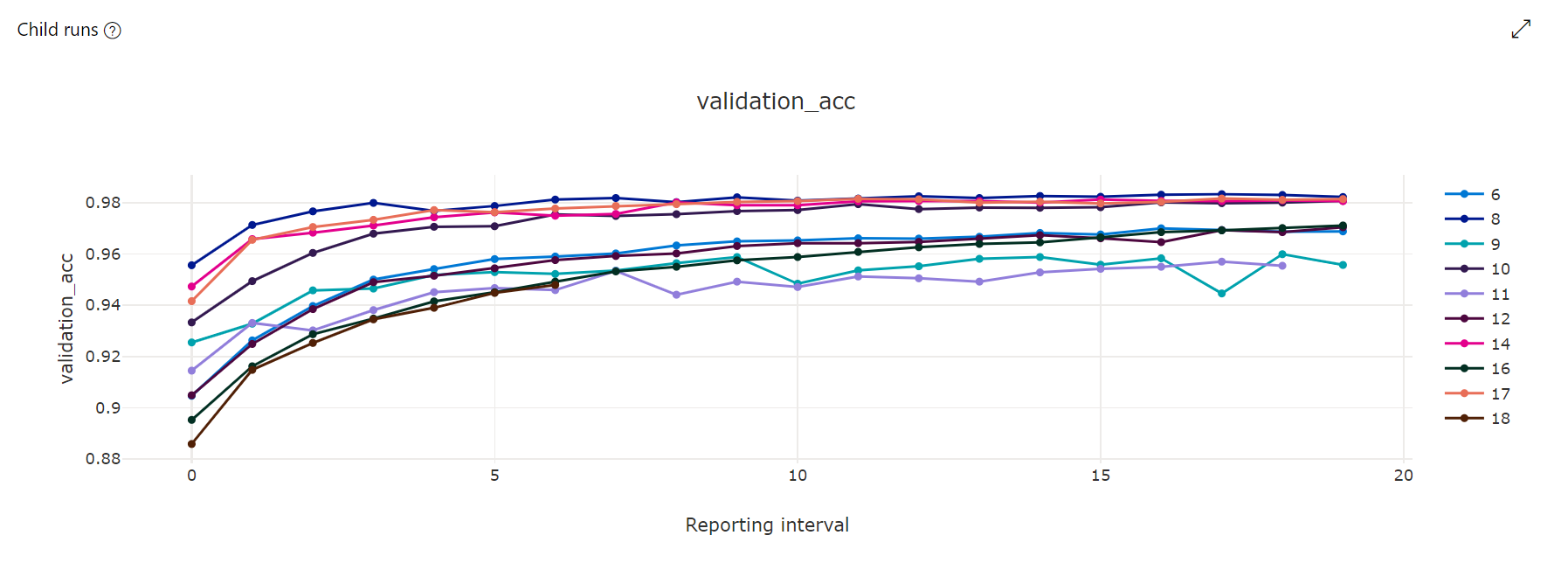• 并行坐标图：此可视化效果显示主要指标性能与单个超参数值之间的关联。 可通过这些方式与图表进行交互：移动轴（单击并拖动轴标签）以及在单个轴上突出显示值（单击并沿单个轴垂直拖动即可突出显示所需范围的值）。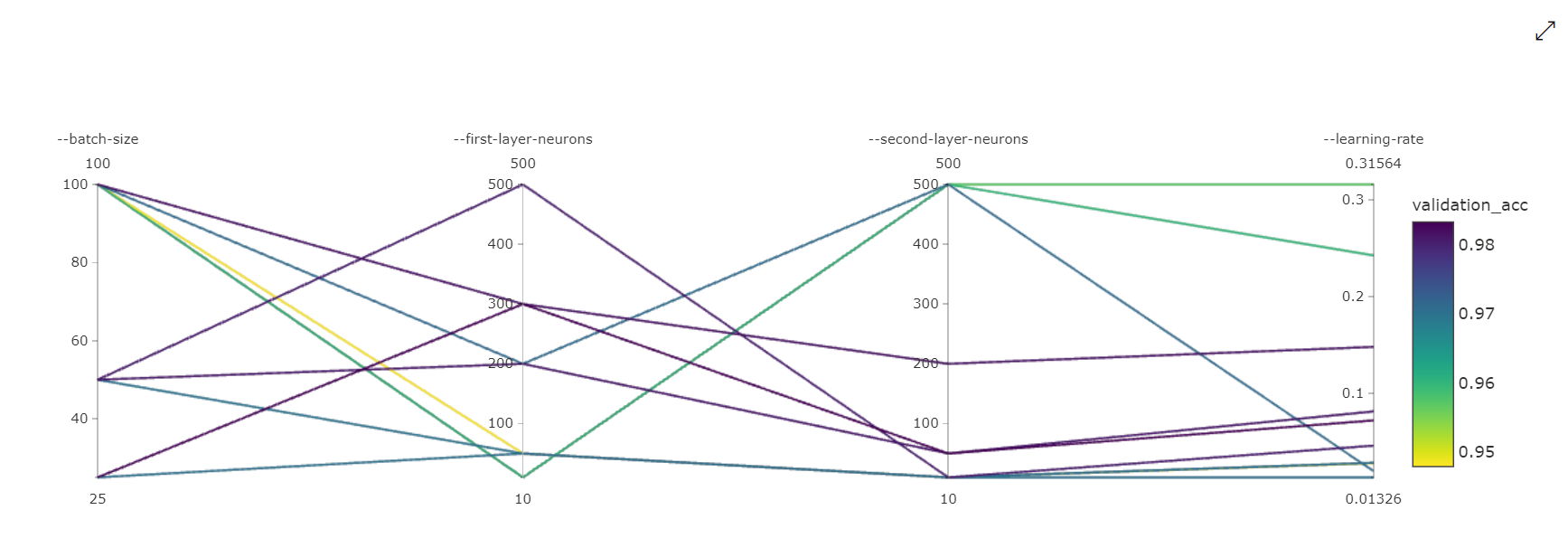• 二维散点图：此可视化效果显示任意两个单独的超参数之间的关联以及它们对应的主要指标值。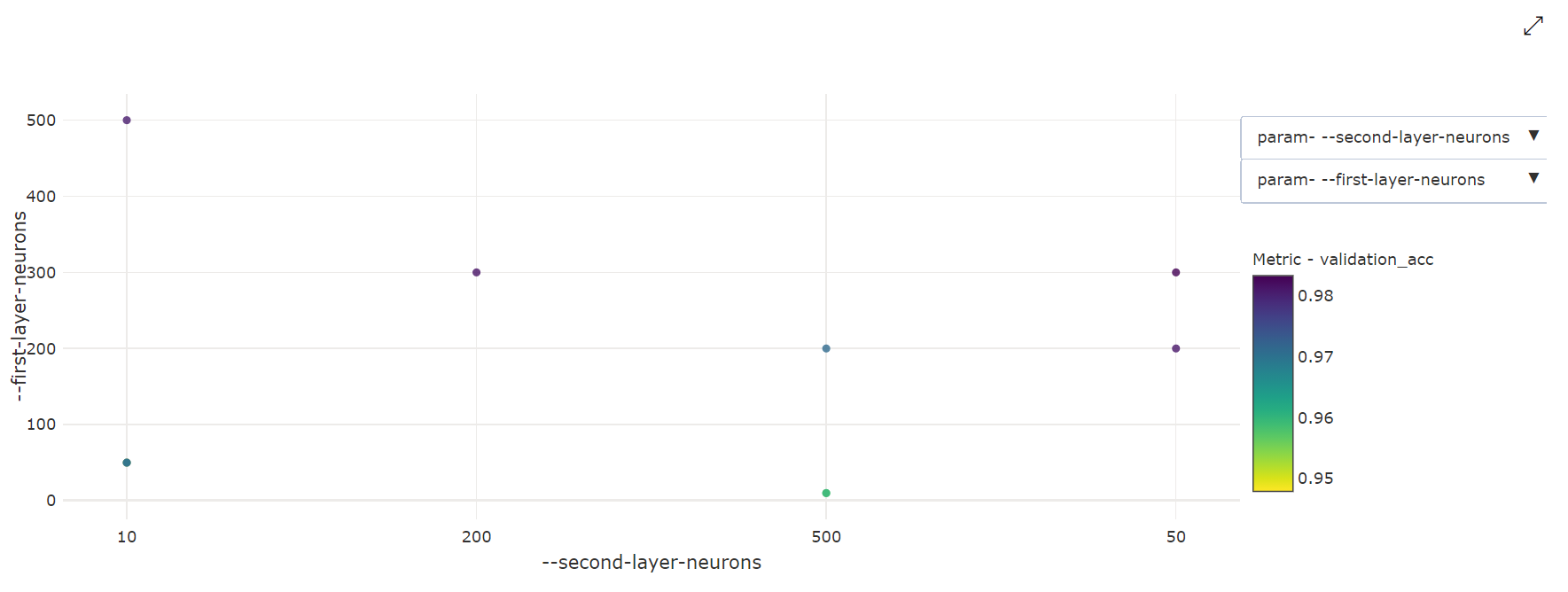• 三维散点图：此可视化效果与二维散点图相同，但可以显示三个超参数维度的关联和主要指标值。 还可以单击并拖动以调整图表，以在三维空间中查看不同的关联。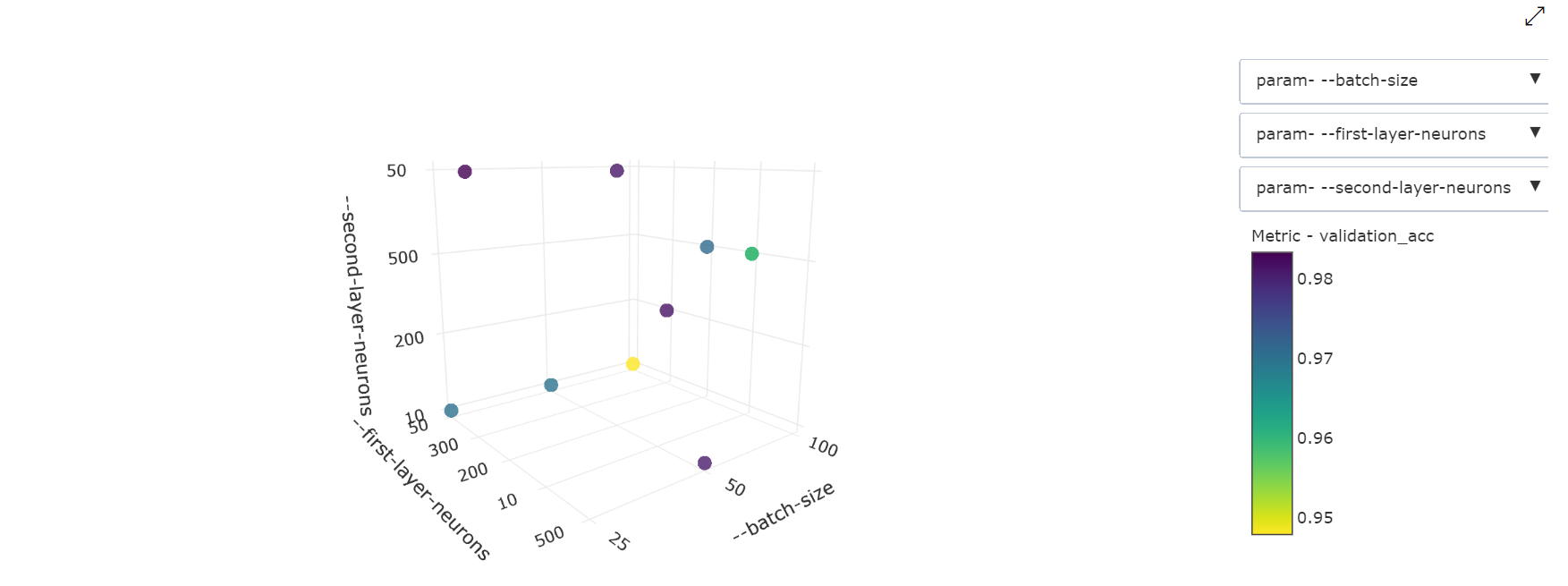### Notebook 小组件

``````from azureml.widgets import RunDetails
RunDetails(hyperdrive_run).show()
``````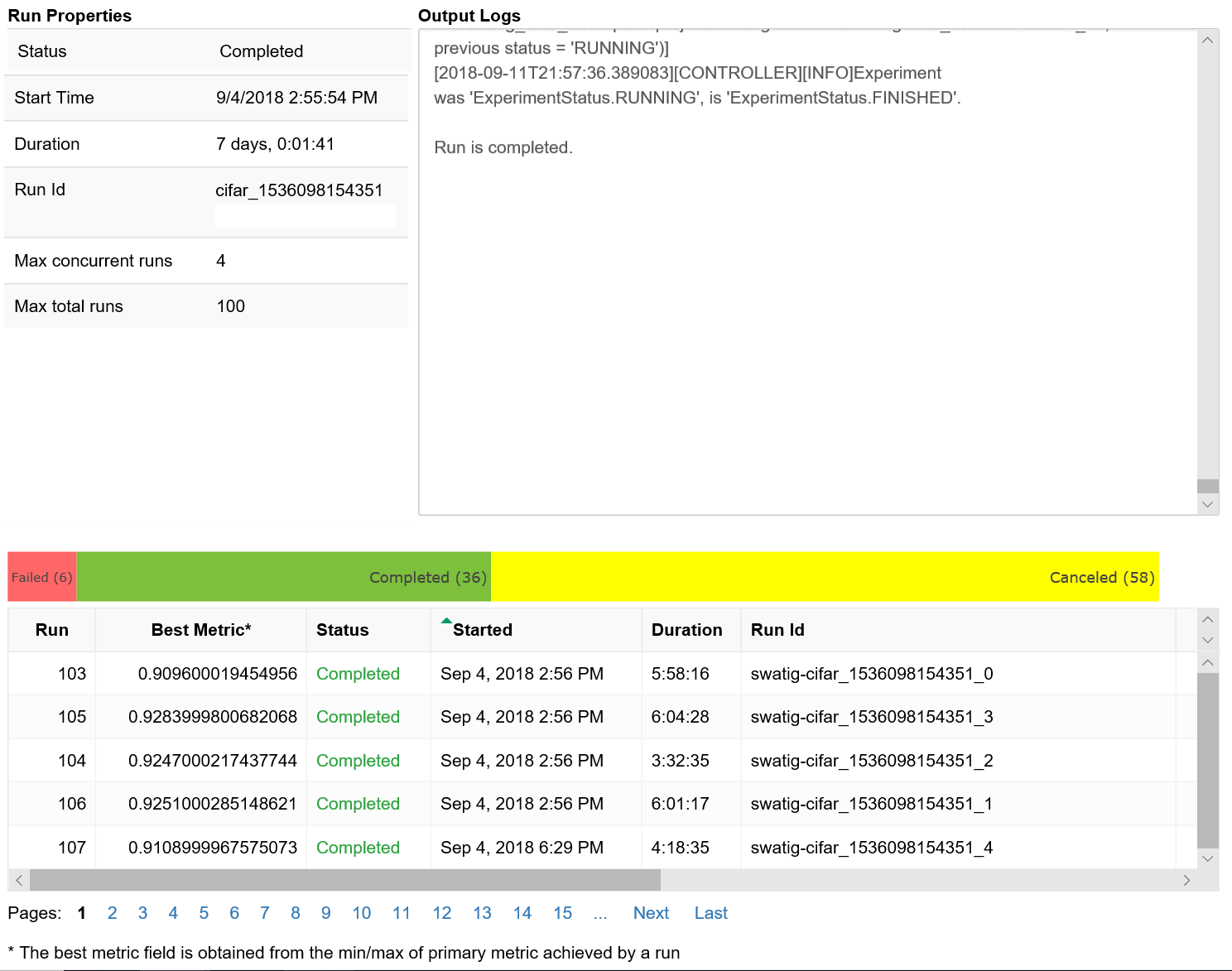## 找到最佳模型

``````best_run = hyperdrive_run.get_best_run_by_primary_metric()
best_run_metrics = best_run.get_metrics()
parameter_values = best_run.get_details()['runDefinition']['Arguments']

print('Best Run Id: ', best_run.id)
print('\n Accuracy:', best_run_metrics['accuracy'])
print('\n learning rate:',parameter_values)
print('\n keep probability:',parameter_values)
print('\n batch size:',parameter_values)
``````